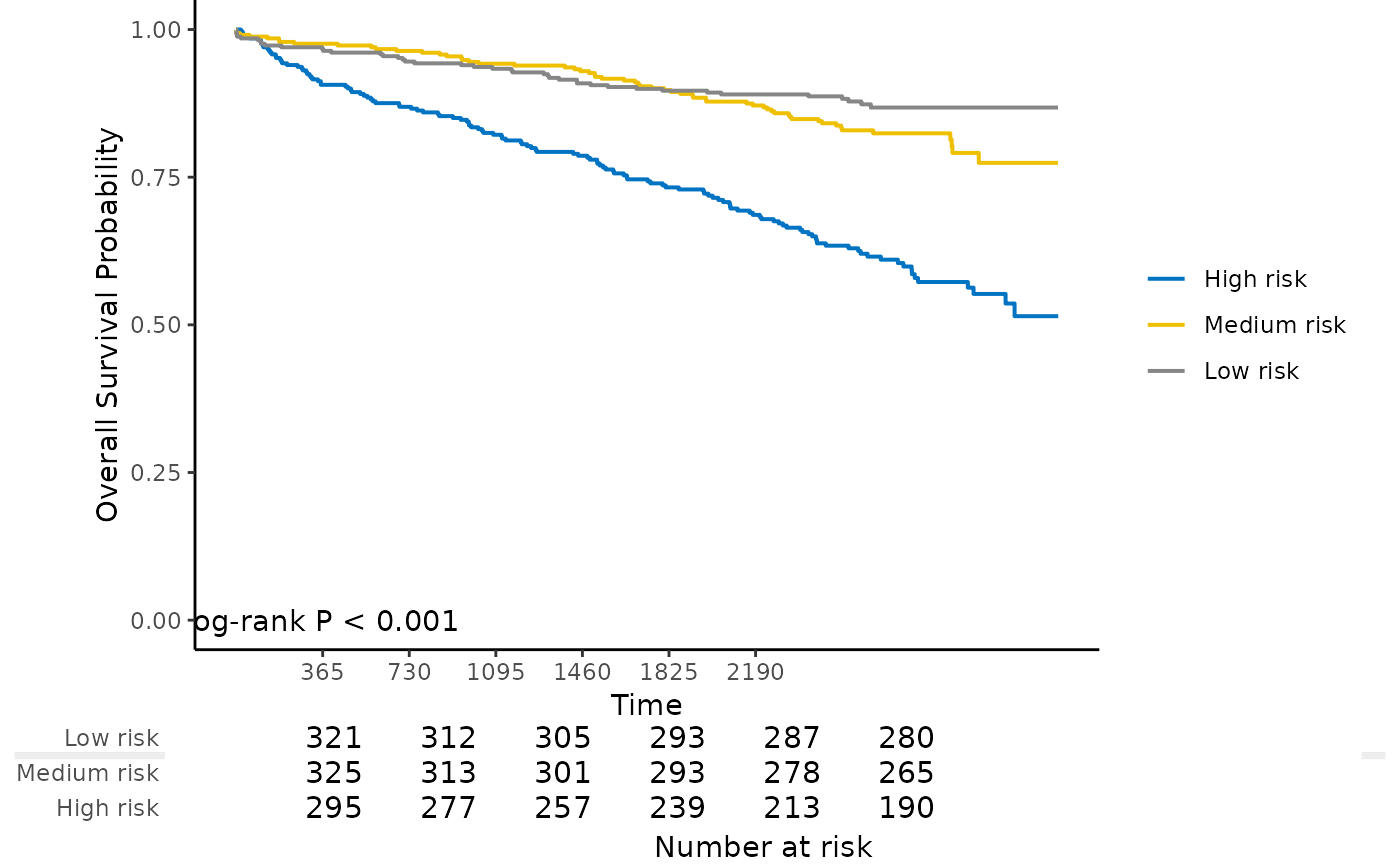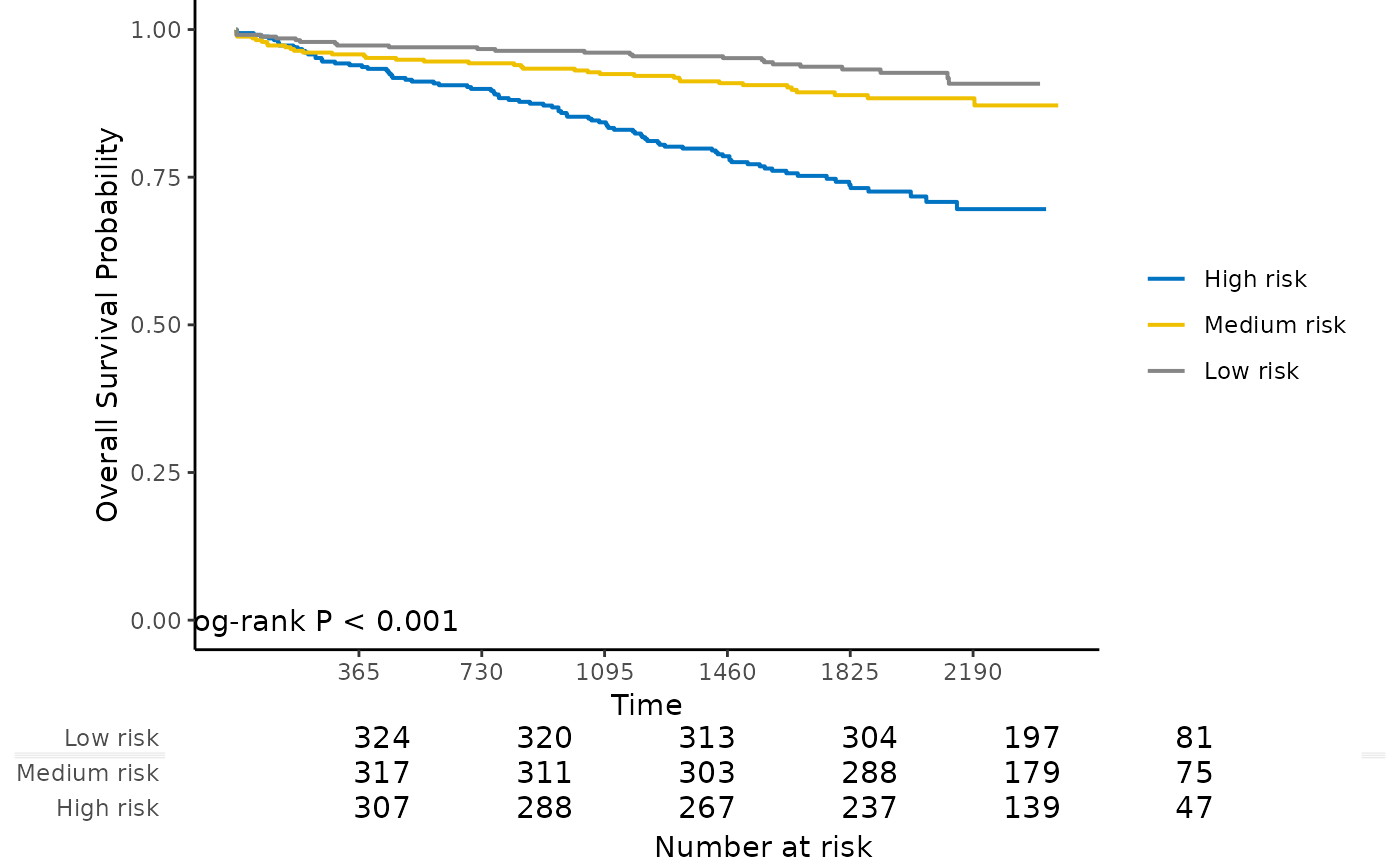Kaplan-Meier plot with number at risk table for internal calibration and external calibration results

kmplot(object, group.name = NULL, time.at = NULL, col.pal = c("JCO",
"Lancet", "NPG", "AAAS"))

## Arguments

object An object returned by calibrate or calibrate_external. Risk group labels. Default is Group 1, Group 2, ..., Group k. Time points to evaluate the number at risk. Color palette to use. Possible values are "JCO", "Lancet", "NPG", and "AAAS". Default is "JCO".

## Examples

data("smart")
# Use the first 1000 samples as training data
# (the data used for internal validation)
x <- as.matrix(smart[, -c(1, 2)])[1:1000, ]
time <- smart$TEVENT[1:1000] event <- smart$EVENT[1:1000]

# Take the next 1000 samples as external calibration data
# In practice, usually use data collected in other studies
x_new <- as.matrix(smart[, -c(1, 2)])[1001:2000, ]
time_new <- smart$TEVENT[1001:2000] event_new <- smart$EVENT[1001:2000]

# Fit Cox model with lasso penalty
fit <- fit_lasso(x, survival::Surv(time, event), nfolds = 5, rule = "lambda.1se", seed = 11)

# Internal calibration
cal.int <- calibrate(
x, time, event,
model.type = "lasso",
alpha = 1, lambda = fit\$lambda,
method = "cv", nfolds = 5,
pred.at = 365 * 9, ngroup = 3
)#> Start fold 1
#> Start fold 2
#> Start fold 3
#> Start fold 4
#> Start fold 5
kmplot(
cal.int,
group.name = c("High risk", "Medium risk", "Low risk"),
time.at = 1:6 * 365
)# External calibration
cal.ext <- calibrate_external(
fit, x, time, event,
x_new, time_new, event_new,
pred.at = 365 * 5, ngroup = 3
)

kmplot(
cal.ext,
group.name = c("High risk", "Medium risk", "Low risk"),
time.at = 1:6 * 365
)